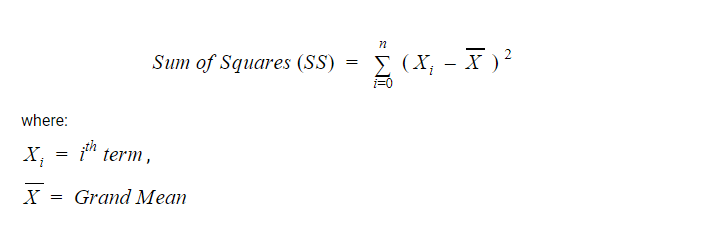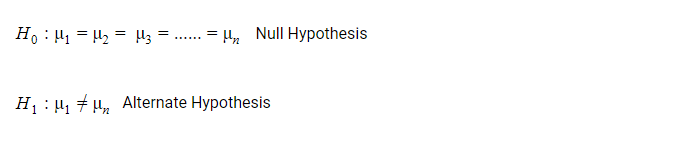• Category
• >Statistics

# ANOVA Test - Definition and Examples

• Ritesh Pathak
• Mar 02, 2021Statistics, being an interdisciplinary field, has several concepts that have found practical applications. These include the Pearson Correlation Coefficient ‘r’, t-test, ANOVA test, etc.

You may have heard about at least one of these concepts, if not, go through our blog on Pearson Correlation Coefficient ‘r’.

In this blog, we will be discussing the ANOVA test.

## History of ANOVA

The history of the ANOVA test dates back to the year 1918. It’s a concept that Sir Ronald Fisher gave out and so it is also called the Fisher Analysis of Variance.

He had originally wished to publish his work in the journal Biometrika, but, since he was on “not so good” terms with its editor Karl Pearson, the arrangement could not take place. So eventually, he settled with the Journal of Agricultural Science.

If you have a little knowledge about the ANOVA test, you would probably know or at least have heard about null vs alternative hypothesis testing. It is an edited version of the ANOVA test. The revamping was done by Karl Pearson’s son Egon Pearson, and Jersey Neyman.

Throughout this blog, we will be discussing Ronald Fisher’s version of the ANOVA test.

We should start with a description of the ANOVA test and then we can dive deep into its practical application, and some other relevant details.

## What does the ANOVA test mean?

The ANOVA, which stands for the Analysis of Variance test, is a tool in statistics that is concerned with comparing the means of two groups of data sets and to what extent they differ.

In simpler and general terms, it can be stated that the ANOVA test is used to identify which process, among all the other processes, is better. The fundamental concept behind the Analysis of Variance is the “Linear Model”.

## Example of ANOVA

An example to understand this can be prescribing medicines.

• Suppose, there is a group of patients who are suffering from fever.

• They are being given three different medicines that have the same functionality i.e. to cure fever.

• To understand the effectiveness of each medicine and choose the best among them, the ANOVA test is used.

You may wonder that a t-test can also be used instead of using the ANOVA test. You are probably right, but, since t-tests are used to compare only two things, you will have to run multiple t-tests to come up with an outcome. While that is not the case with the ANOVA test.

That is why the ANOVA test is also reckoned as an extension of t-test and z-tests.

## Terminologies in ANOVA Test

There are few terms that we continuously encounter or better say come across while performing the ANOVA test. We have listed and explained them below:

### 1. Means(Grand and Sample)

As we know, a mean is defined as an arithmetic average of a given range of values. In the ANOVA test, there are two types of mean that are calculated: Grand and Sample Mean.

A sample mean (μn) represents the average value for a group while the grand mean (μ) represents the average value of sample means of different groups or mean of all the observations combined.### 2. F-Statistic

The statistic which measures the extent of difference between the means of different samples or how significantly the means differ is called the F-statistic or F-Ratio. It gives us a ratio of the effect we are measuring (in the numerator) and the variation associated with the effect (in the denominator).

The formula given to calculate the F-Ratio is:Since we use variances to explain both the measure of the effect and the measure of the error, F is more of a ratio of variances. The value of F can never be negative.

• When the value of F exceeds 1 it means that the variance due to the effect is larger than the variance associated with sampling error; we can represent it as:

When F>1, variation due to the effect > variation due to error

• If F<1, it means variation due to effect < variation due to error

• When F = 1 it means variation due to effect = variation due to error. This situation is not so favorable.

### 3. Sums of Squares

In statistics, the sum of squares is defined as a statistical technique that is used in regression analysis to determine the dispersion of data points. In the ANOVA test, it is used while computing the value of F.

As the sum of squares tells you about the deviation from the mean, it is also known as variation.

The formula given to calculate the sum of squares is:While calculating the value of F, we need to find SSTotal that is equal to the sum of SSEffect and SSError

SSTotal = SSEffect + SSError

### 4. Degrees of Freedom (Df)

Degrees of Freedom refers to the maximum numbers of logically independent values that have the freedom to vary in a data set.### 5. Mean Squared Error (MSE)

The Mean Squared Error tells us about the average error in a data set. To find the mean squared error, we just divide the sum of squares by the degrees of freedom.### 6. Hypothesis (Alternate and Null)

Hypothesis, in general terms, is an educated guess about something around us. When we are given a set of data and are required to predict, we use some calculations and make a guess. This is all a hypothesis.

In the ANOVA test, we use Null Hypothesis (H0) and Alternate Hypothesis (H1). The Null Hypothesis in ANOVA is valid when the sample means are equal or have no significant difference.

The Alternate Hypothesis is valid when at least one of the sample means is different from the other.### 7. Group Variability (Within-group and Between-group)

To understand group variability, we should know about groups first. In the ANOVA test, a group is the set of samples within the independent variable.

There are variations among the individual groups as well as within the group. This gives rise to the two terms: Within-group variability and Between-group variability.

• When there is a big variation in the sample distributions of the individual groups, it is called between-group variability.

• On the other hand, when there are variations in the sample distribution within an individual group, it is called Within-group variability.

## Types of ANOVA Test

The ANOVA test is generally done in three ways depending on the number of Independent Variables (IVs) included in the test. Sometimes the test includes one IV, sometimes it has two IVs, and sometimes the test may include multiple IVs.

We have three known types of ANOVA test:

1. One-Way ANOVA

2. Two-Way ANOVA

3. N-Way ANOVA (MANOVA)

### One-Way ANOVA

One-way ANOVA is generally the most used method of performing the ANOVA test. It is also referred to as one-factor ANOVA, between-subjects ANOVA, and an independent factor ANOVA. It is used to compare the means of two independent groups using the F-distribution.

Two carry out the one-way ANOVA test, you should necessarily have only one independent variable with at least two levels. One-way ANOVA does not differ much from t-test.

Example where one-way ANOVA is used

Suppose a teacher wants to know how good he has been in teaching with the students. So, he can split the students of the class into different groups and assign different projects related to the topics taught to them.

He can use one-way ANOVA to compare the average score of each group. He can get a rough understanding of topics to teach again. However, he won’t be able to identify the student who could not understand the topic.

### Two-way ANOVA

Two-way ANOVA is carried out when you have two independent variables. It is an extension of one-way ANOVA. You can use the two-way ANOVA test when your experiment has a quantitative outcome and there are two independent variables.

Two-way ANOVA is performed in two ways:

1. Two-way ANOVA with replication: It is performed when there are two groups and the members of these groups are doing more than one thing. Our example in the beginning can be a good example of two-way ANOVA with replication.

1. Two-way ANOVA without replication: This is used when you have only one group but you are double-testing that group. For example, a patient is being observed before and after medication.

Assumptions for Two-way ANOVA

• The population must be close to a normal distribution.

• Samples must be independent.

• Population variances must be equal.

• Groups must have equal sample sizes.

### N-way ANOVA (MANOVA)

When we have multiple or more than two independent variables, we use MANOVA. The main purpose of the MANOVA test is to find out the effect on dependent/response variables against a change in the IV.

• Does the change in the independent variable significantly affect the dependent variable?

• What are interactions among the dependent variables?

• What are interactions between independent variables?

MANOVA is advantageous as compared to ANOVA because it allows you to test multiple dependent variables and protects from Type I errors where we ignore a true null hypothesis.

Also Read: Crash Course in Statistics

## Real-world application of ANOVA test

Suppose medical researchers want to find the best diabetes medicine and they have to choose from four medicines. They can choose 20 patients and give them each of the four medicines for four months.

The researchers can take note of the sugar levels before and after medication for each medicine and then to understand whether there is a statistically significant difference in the mean results from the medications, they can use one-way ANOVA.

The type of medicine can be a factor and reduction in sugar level can be considered the response. Researchers can then calculate the p-value and compare if they are lower than the significance level.

If the results reveal that there is a statistically significant difference in mean sugar level reductions caused by the four medicines, the post hoc tests can be run further to determine which medicine led to this result.